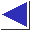# comp.lang.c FAQ list ·Question 10.1

Q: I'm trying to define a few simple little function-like macros such as

```	#define square(x) x * x
```
but they're not always working.

A: There are three important rules to remember when defining function-like macros:

1. The macro expansion must always be parenthesized to protect any lower-precedence operators from the surrounding expression. Given the (incorrect) square() macro above, the invocation
```	1 / square(n)
```
would expand to
```	1 / n * n
```
(which evaluates as (1 / n) * n), while what you want is
```	1 / (n * n)
```
(In this case, the problem is one of associativity rather than precedence, but the effect is the same.)
2. Within the macro definition, all occurrences of the parameters must be parenthesized to protect any low-precedence operators in the actual arguments from the rest of the macro expansion. Again given the square() macro above, the invocation
```	square(n + 1)
```
would expand to
```	n + 1 * n + 1
```
But what you want is
```	(n + 1) * (n + 1)
```
3. If a parameter appears several times in the expansion, the macro may not work properly if the actual argument is an expression with side effects. Yet again given the square() macro above, the invocation
```	square(i++)
```
would expand to
```	i++ * i++
```
which is undefined (see question 3.2).

The proper definition of a square macro, to comply with rules 1 and 2 above, is

```	#define square(x) ((x) * (x))
```
Complying with rule 3 is harder. Sometimes, careful exploitation of the short-circuiting behavior of the &&, ||, or ?: operators (see question 3.6) can arrange that a parameter which appears several times is guaranteed to be evaluated exactly once. Sometimes, the macro is just documented as being unsafe, and callers must remember not to use it on arguments with side effects. Other times, it may be advisable not to compose a function-like macro if it can't be made safe.

(As a stylistic convention, macros are often defined with capitalized or all-upper-case names, to make it obvious that they are macros. It may be acceptable to define a function-like macro with an all-lower-case name, if it truly simulates a function, but only if it complies with all three rules above. Since the squaring macro we've been discussing does not, it should be defined as something like

```	#define Square(x) ((x) * (x))	/* UNSAFE */
```
if it is to be used at all.)

References: K&R1 Sec. 4.11 p. 87
K&R2 Sec. 4.11.2 p. 90
H&S Secs. 3.3.6,3.3.7 pp. 49-50
CT&P Sec. 6.2 pp. 78-80